## Wednesday, August 13, 2014

### Mathematical equations of love, heart, penis and the boomerang

Love

Love is complicated. But the mathematics of it is very simple:
- It starts with "I love you" where "I love" is a constant, and "you" is a variable.
- Later on, it is:  1 + 1 = 1
- And later still:  1 + 1 >= 3
Any questions?

Now, let us explore the mechanics of love.

Heart

Love comes from the heart. The mathematical equation of the heart is:To see the above graph, go to WolframAlpha website
http://www.wolframalpha.com/
At the input area, type in:
(y - 0.75|x|)^2 + (0.75x)^2 = 1
And you'll see the heart curve.

You get a slightly different shape of the heart by changing the value of 0.75. Have a try at 0.6 or 0.9 or other values. I find 0.75 more aethetically pleasing.

Actually, it just strikes me that the following parametrised equation
(y - a|x|^b)^2 + (cx)^2 = d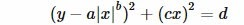(where you can set the values of the parameters  a, b, c and d)
can draw just about any heart shaped curve that one can imagine (and more) ... I'm claiming this parametrised equation as Paul Ma's Heart Equation.

So far, no one has disputed my claim in the math.stackexchange forum:
http://math.stackexchange.com/questions/902120/is-there-a-name-to-this-equation-y-axb2-cx2-d

By setting  a=0.75,  b=1,  c=0.75  d=1  into Paul's Heart Equation, it becomes the previously mentioned heart curve.

If you set  a=1,  b=0.5,  c=1  d=4,  you'll produce:
(y - |x|^0.5)^2 + x^2 = 4
Type the above into the WolframAlpha input area and you'll see that its heart is pretty good looking too:How about you have a go at various other values of the parameters  a, b, c, d?  Examples are:
a=0.5,  b=0.5,  c=0.7  d=0.5
a=0.6,  b=(2/3),  c=0.8  d=0.9

If you discover other good sets of values to use, I would be interested to hear from you.

Boomerang

Now, when you give out love, love always comes back to you. Hence you expect the mathematical equation of a boomerang to be similar to that of a heart, right?  Indeed it is.  Set  a=0.5,  b=1,  c=0.13,  d=1  in Paul's Heart Equation to produce:
(y - 0.5|x|)^2 + (0.13x)^2 = 1
Type the above into WolframAlpha and you'll getDoes love make the world go round?

Well, the heart certainly makes the world go round. Take a look at Paul's Heart Equation again:Let  a=0, b=any, c=1, d=1  and it becomes a perfect circle !
y^2 + x^2 = 1But in a real world nothing is ever so perfect.  There are always pits and bumps.
Set  a=1, b=0.5, c=1, d=500  and you'll get:
(y - |x|^0.5)^2 + x^2 = 500Penis

Of course, you can't talk about love without mentioning the penis. The mathematical equation of a penis is:
y = |sin(x)| + 5*exp(-x^100)*cos(x)  from -3 to 3
Type the above into WolframAlpha to produceThe Bum

This is covered in my blog From Golden Ratio to golden arse
http://onemanadreaming.blogspot.com.au/2014/04/from-golden-ratio-to-golden-arse.html

Breast

To be discussed later   :-)   ... watch this blog   :-)

Post Script

There are other equations for the penis and heart.

An equation for the penis in polar form:
y = Cos(x) + Cos(2x)  polarAnother equation of the heart:
(y^2 + x^2 - 1)^3 - (x^2)*(y^3) = 0Here is another one:
x^2 + (y - (2(x^2+|x|-6)) / (3(x^2+|x|+2)))^2 = 36Using 2 equations:
y = (1-(|x|-1)^2)^0.5  and  y = -3(1-(|x|/2)^0.5)^0.5  from -2 to 2In polar form:
y = x  polar  (x from -1.5pi to 1.5pi)Another one in polar form:
y = (sin(x) sqrt(|cos(x)|) / (sin(x) + 1.4)) - 2sin(x) + 2  polar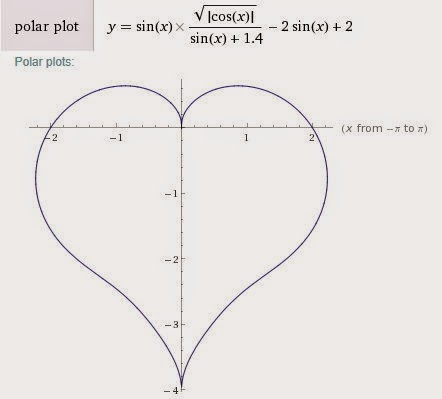And another one in polar form - this equation has a name, called a Cardioid:
y = 1 - sin(x)  polar
Its corresponding Cartesian equation is:
(x^2 + y^2 + y)^2 = x^2 + y^2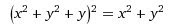In 3D

(x^2 + 2.25y^2 + z^2 - 1)^3 - (x^2)(z^3) - 0.1125(y^2)(z^3) = 0
(called Taubin heart surface)Share

Please visit my main page at http://mntviews.blogspot.com/

#### 12 comments:

1.Amazing!! Completely fall in love with that!! <3 I left my own heart as well..;)

2.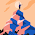Funny way of presenting graphicsEquations using images. Keep it up. thanks for sharing

1.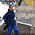Thank you for your comment :-)

3.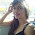4.what about the 'poo' curve?

5."Of course, you can't talk about love without mentioning the penis."

I don't think so.

1.6.What about the equation for a 3d penis

1.I'll leave it as an exercise for you :-)

2.Just parameterize and rotate the peen about the y axis.

7.Put a=1, b=-0.5 c=1 d=4 you will get testicals

8.what is a penis? someone please tell meDonations are welcome to support the creation of more interesting articles in this blog  :-)